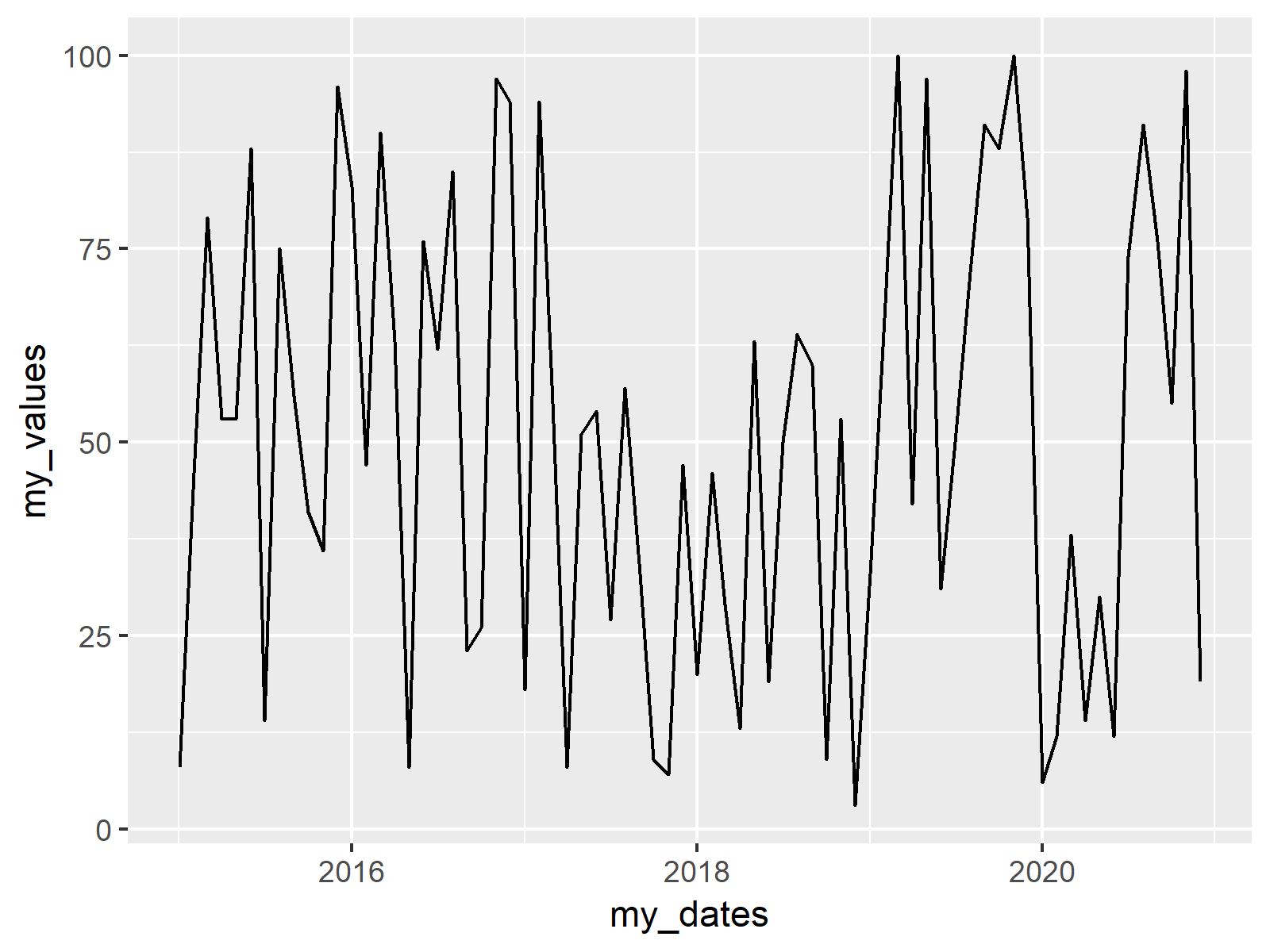# Draw Vertical Line to X-Axis of Class Date in ggplot2 Plot in R (Example)

In this tutorial you’ll learn how to add a geom_vline to a ggplot2 plot with dates as x-axis in the R programming language.

The tutorial will contain one examples for drawing vertical lines. More precisely, the content of the post is structured as follows:

Let’s dive right into the examples.

## Creating Exemplifying Data

In the example of this R programming tutorial, we’ll use the following R data frame as basement:

```set.seed(1510)                                                        # Create data frame
data <- data.frame(my_dates = seq(as.Date(0, origin="2015-01-01"),
length = 72,
by = "1 month"),
my_values = sample(1:100, 72, replace = TRUE))
#     my_dates my_values
# 1 2015-01-01         8
# 2 2015-02-01        46
# 3 2015-03-01        79
# 4 2015-04-01        53
# 5 2015-05-01        53
# 6 2015-06-01        88```

Our example data contains 72 rows and two columns. The variable my_dates has the class Date and the column my_values consists of random numeric values.

If we want to draw graphics with the ggplot2 package, we also need to install and load ggplot2:

```install.packages("ggplot2")                                           # Install ggplot2

Now, we can draw a basic ggplot2 line plot without any vertical lines as follows:

```ggp <- ggplot(data, aes(my_dates, my_values)) +                       # Create basic ggplot
geom_line()
ggp                                                                   # Draw basic ggplot```Figure 1: ggplot2 Line Plot with Default Specifications.

Figure 1 illustrates the output of the previous R code – A basic line plot showing values corresponding to dates on the x-axis.

## Example: Adding geom_vline at Specific Dates

If we want to add vertical lines at some specific dates to our plot, we first need to specify the dates and their positions in our data:

```dates_vline <- as.Date(c("2015-11-01", "2018-03-01"))                 # Define positions of vline
dates_vline <- which(data\$my_dates %in% dates_vline)```

Now, we can add vertical lines with the geom_vline function to our plot:

```ggp +                                                                 # Draw vlines to plot
geom_vline(xintercept = as.numeric(data\$my_dates[dates_vline]),
col = "red", lwd = 2)```Figure 2: ggplot2 Line Plot with Vertical Lines at Date Positions.

Figure 2 shows the result: A ggplot2 line plot with two vertical lines.

## Video, Further Resources & Summary

If you need more explanations on the R code of this tutorial, you might watch the following video of my YouTube channel. I’m explaining the R codes of this article in the video:

Furthermore, you might want to have a look at the other articles of my homepage. Some tutorials can be found below.

Summary: In this tutorial, I showed how to get a vertical geom_vline to an x-axis of class date in R programming. Don’t hesitate to let me know in the comments, in case you have further questions and/or comments.

Subscribe to the Statistics Globe Newsletter